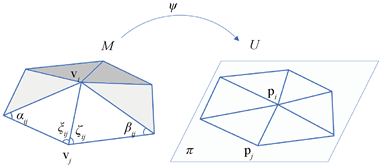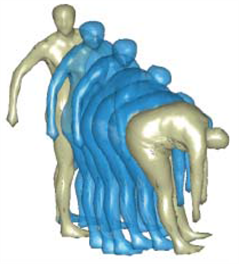# 基于离散微分几何的3D网格模型分析进展综述Review on 3D Mesh Model Analysis Based on the Discrete Differential Geometry

DOI: 10.12677/MET.2019.85041, PDF, HTML, XML, 下载: 428  浏览: 1,406

Abstract: The analysis of 3D models has become a hot topic in computer graphics research in recent years. Aiming at the application problems of mesh denoising and smoothing, mesh parameterization and non-rigid registration in the field of discrete differential geometry, this paper reviews the literature on the application of discrete differential geometry theory in analysis, mathematical modeling and algorithm design in recent years, and introduces the research progress of discrete differential geometry in the field of 3D model analysis. The difficulties and possible research directions in the future are summarized.

1. 引言

2. 离散微分几何的理论框架

2.1. 基本概念

2.2. 算子

$\nabla u\left(v\right)=\underset{i}{\sum }{u}_{i}\nabla {\delta }_{i}\left(v\right).$ (1)

$\nabla \cdot w\left({v}_{i}\right):=\underset{{f}_{j}\in {N}_{f}\left({v}_{i}\right)}{\sum }{A}_{{f}_{j}}w\left({f}_{j}\right)\cdot {\nabla {\delta }_{i}|}_{{f}_{j}},$ (2)

$\Delta u=\nabla \cdot \nabla u=\underset{j\in {i}^{\ast }}{\sum }{\omega }_{ij}\left({u}_{i}-{u}_{j}\right),$ (3)

$\left(\Delta -\frac{\partial }{\partial t}\right)u\left(x,t\right)=0.$ (4)

${h}_{t}\left(x,y\right)=\underset{i=0}{\overset{\infty }{\sum }}{\text{e}}^{-{\lambda }_{i}t}{\varphi }_{i}\left(x\right){\varphi }_{i}\left(y\right),$ (5)

3. 基于离散微分几何的3D模型分析

3.1. 网格去噪光滑

$\Delta u=-Ku,$ (6)

${u}^{N}=h{\left(K\right)}^{N}u.$ (7)

3.2. 网格参数化

(1) Dirichlet能量方法的网格参数化

$E\left({v}_{i}\right)=\lambda {E}_{A}\left({v}_{i}\right)+\mu {E}_{\chi }\left({v}_{i}\right),$ (8)

${E}_{A}\ge \frac{1}{2}\underset{M}{\int }{f}_{u}×{f}_{v}\text{d}u\text{d}v$ .

${E}_{A}\left({x}_{i}\right)=\underset{j\in {i}^{*}}{\sum }\mathrm{cot}{\alpha }_{ij}{‖{v}_{i}-{v}_{j}‖}^{2}.$ (9)

${E}_{\chi }$ 为欧拉示性数的变形能量，定义为：

${E}_{\chi }\left({x}_{i}\right)=\underset{j\in {i}^{*}}{\sum }\frac{\mathrm{cot}{\xi }_{ij}+\mathrm{cot}{\zeta }_{ij}}{{‖{v}_{i}-{v}_{j}‖}^{2}}{‖{p}_{i}-{p}_{j}‖}^{2},$ (10)Figure 1. A ring on a mesh mapped to a ring on a plane domain

$\frac{\partial {E}_{A}}{\partial {p}_{i}}=\underset{j\in {i}^{*}}{\sum }\left(\mathrm{cot}{\alpha }_{ij}+\mathrm{cot}{\beta }_{ij}\right)|{p}_{i}-{p}_{j}|=0$ (11)

$\frac{\partial {E}_{\chi }}{\partial {p}_{i}}=\underset{j\in {i}^{*}}{\sum }\frac{\left(\mathrm{cot}{\xi }_{ij}+\mathrm{cot}{\zeta }_{ij}\right)|{p}_{i}-{p}_{j}|}{{‖{v}_{i}-{v}_{j}‖}^{2}}=0$ (12)

(2) Circle packing的网格参数化

Thurston首先提出了用一系列变化的圆来近似从平面到单位圆盘的黎曼映射，从而实现网格到平面的保角映射  。文献  提出一种新的构造任意拓扑面网格到平面的离散共形映射的方法。该方法是基于圆模式(Circle packing)，即每个三角面与一个圆相对应，按照预设的夹角来调整圆的半径来达到保角映射的目的。该方法相对于调和映射有两方面的优势：1) 支持自然边界条件和规定的曲率控制边界形状的边界；2) 该解是基于作为函数的凸能量，用简单的显式表达式表示对数半径变量梯度和Hessian矩阵，因而更为稳健和高效。

$\frac{\text{d}{\gamma }_{i}}{\text{d}t}=\left({\stackrel{˜}{K}}_{{v}_{i}}-{K}_{{v}_{i}}\right){\gamma }_{i},$ (13)

$F\left(u\right)=-{\int }_{0}^{u}\underset{i}{\sum }\left({\stackrel{˜}{K}}_{{v}_{i}}-{K}_{{v}_{i}}\right)\text{d}{u}_{i}.$ (14)

3.3. 非刚性配准

3D刚性配准问题是指同一个实体在不同坐标系下采集得到的两个3D模型，经过刚体变换到同一个坐标系的同样姿态。这类算法是目标识别，误差估计算法的上游算法，具有广泛的应用意义，实现的算法也非常多。一般的分类如图2Figure 2. Classification of registration algorithms

$\left({\mathcal{D}}^{*},{\gamma }^{*}\right)=\underset{\mathcal{D}\in SO\left(3\right),\gamma \in \Gamma }{\mathrm{arg}\mathrm{min}}\stackrel{˜}{d}\left({S}_{1},\mathcal{D}{S}_{2}\circ \gamma \right),$ (15)Figure 3. Transitional deformation between two models measured by geodesic lines, extracted from Figure 7.2(c) in reference 

$L\left(\Theta \right)={\int }_{0}^{1}{〈{\nabla }_{\tau }\Theta \left(\tau \right),{\nabla }_{\tau }\Theta \left(\tau \right)〉}^{1/2}\text{d}\tau .$ (16)

${\Theta }^{*}=\underset{\left(D,\gamma \right)\in SO\left(3\right)×\Gamma }{\mathrm{arg}\mathrm{min}}\left\{\underset{\begin{array}{l}\Theta :\left[0,1\right]\to S,\\ \Theta \left(0\right)={S}_{1},\\ \Theta \left(1\right)=D\left({S}_{1}\circ \gamma \right)\end{array}}{\mathrm{min}}L\left(\Theta \right)\right\}.$ (17)

4. 总结

NOTES

*通讯作者。

  Laga, H., et al. (2018) 3D Shape Analysis: Fundamentals, Theory, and Applications. Wiley, Hoboken, 368. https://doi.org/10.1002/9781119405207  Meyer, M. (2002) Discrete Differential-Geometry Operator for Triangu-lated 2-Manifolds. Visualization & Mathematics, 3, 35-57. https://doi.org/10.1007/978-3-662-05105-4_2  Sun, J., Ovsjanikov, M. and Guibas, L. (2010) A Concise and Provably Informative Multi-Scale Signature Based on Heat Diffusion. Computer Graphics Forum, 28, 1383-1392. https://doi.org/10.1111/j.1467-8659.2009.01515.x  Benguria, R.D., Brandolini, B. and Chiacchio, F. (2019) A Sharp Estimate for Neumann Eigenvalues of the Laplace-Beltrami Operator for Domains in a Hemisphere. Communications in Contemporary Mathematics. https://doi.org/10.1142/S0219199719500184  Qi, C.R., et al. (2017) PointNet++: Deep Hierarchical Feature Learning on Point Sets in a Metric Space.  Wang, Y., et al. (2018) Dynamic Graph CNN for Learning on Point Clouds.  Han, Z., et al. (2017) Mesh Convolutional Restricted Boltzmann Machines for Unsupervised Learning of Fea-tures with Structure Preservation on 3-D Meshes. IEEE Transactions on Neural Networks & Learning Systems, 28, 2268-2281. https://doi.org/10.1109/TNNLS.2016.2582532  Minakshisundaram, S. and Pleijel, Å. (1949) Some Properties of the Eigenfunctions of the Laplace-Operator on Riemannian Manifolds. Canadian Journal of Mathematics, 3, 242-256. https://doi.org/10.4153/CJM-1949-021-5  Bott, R. and Tu, L.W. (2009) Differential Forms in Algebraic Topology. Graduate Texts in Mathematics Book Series (GTM, Volume 82). Springer, New York.  Desbrun, M., et al. (1999) Implicit Fairing of Irregular Meshes Using Diffusion and Curvature Flow. ACM SIGGRAPH Proceedings, Los An-geles, 28 July 2019, 317-324. https://doi.org/10.1145/311535.311576  Vaxman, A., Ben-Chen, M. and Gotsman, C. (2010) A Multi-Resolution Approach to Heat Kernels on Discrete Surfaces. ACM Transactions on Graphics, 29, 1-10. https://doi.org/10.1145/1778765.1778858  Field, D.A. (2010) Laplacian Smoothing and Delaunay Triangulations. International Journal for Numerical Methods in Biomedical Engineering, 4, 709-712. https://doi.org/10.1002/cnm.1630040603  Taubin, G. (1995) A Signal Processing Approach to Fair Surface Design. Proceedings of the 22nd Annual Conference on Computer Graphics and Interactive Techniques, Los Angeles, 6-11 August 1995, 351-358. https://doi.org/10.1145/218380.218473  Ohtake, Y., Belyaev, A. and Bogaevski, I. (2001) Mesh Regularization and Adaptive Smoothing. Computer Aided Design, 33, 789-800. https://doi.org/10.1016/S0010-4485(01)00095-1  Yadav, S.K., Reitebuch, U. and Polthier, K. (2017) Robust and High Fidelity Mesh Denoising. IEEE Transactions on Visualization & Computer Graphics, 99, 2304-2310. https://doi.org/10.1109/TVCG.2018.2828818  Devito, Z., et al. (2017) Opt: A Domain Specific Language for Non-Linear Least Squares Optimization in Graphics and Imaging. ACM Transactions on Graphics, 36, 1-27. https://doi.org/10.1145/3132188  Taime, A., Saaidi, A. and Satori, K. (2015) Comparative Study of Mesh Simplification Algorithms. Proceedings of the Mediterranean Conference on Information & Communication Technologies, Saïdia, 7-9 May 2015, 287-295. https://doi.org/10.1007/978-3-319-30301-7_30  Dym, N., Shtengel, A. and Lipman, Y. (2015) Homotopic Morphing of Planar Curves. Computer Graphics Forum, 34, 239-251. https://doi.org/10.1111/cgf.12712  Liu, X., et al. (2010) Constrained Fairing for Meshes. Computer Graphics Fo-rum, 20, 115-123. https://doi.org/10.1111/1467-8659.00483  Zheng, Y., et al. (2011) Bilateral Normal Filtering for Mesh Denoising. IEEE Transactions on Visualization & Computer Graphics, 17, 1521-1530. https://doi.org/10.1109/TVCG.2010.264  Zhang, J., et al. (2018) Static/Dynamic Filtering for Mesh Geometry. IEEE Transactions on Visualization & Computer Graphics, 25, 1774-1787.  Yuan, G. and Sheng, Z. (2008) Monotone Finite Volume Schemes for Diffusion Equations on Polygonal Meshes. Journal of Computational Physics, 227, 6288-6312. https://doi.org/10.1016/j.jcp.2008.03.007  Seo, S., Chung, M.K. and Vorperian, H.K. (2010) Heat Kernel Smoothing Using Laplace-Beltrami Eigenfunctions. In: International Conference on Medical Image Computing & Computer-Assisted Intervention, Springer, Beijing, 505-512. https://doi.org/10.1007/978-3-642-15711-0_63  Wei, M., et al. (2019) Mesh Denoising Guided by Patch Normal Co-Filtering via Kernel Low-Rank Recovery. IEEE Transactions on Visualization and Computer Graphics, 25, 2910-2926. https://doi.org/10.1109/TVCG.2018.2865363  Tao, L., et al. (2016) Secondary Laplace Operator and Generalized Giaquinta-Hildebrandt Operator with Applications on Surface Segmentation and Smoothing. Computer-Aided Design, 70, 56-66. https://doi.org/10.1016/j.cad.2015.07.009  Alexa, M. and Wardetzky, M. (2011) Discrete Laplacians on General Polygonal Meshes. ACM Transactions on Graphics, 30, Article No. 102. https://doi.org/10.1145/2010324.1964997  Manzini, G., Russo, A. and Sukumar, N. (2014) New Perspectives on Polygonal and Polyhedral Finite Element Methods. Mathematical Models & Methods in Applied Sciences, 24, 1665-1699. https://doi.org/10.1142/S0218202514400065  Carl, W. (2016) A Laplace Operator on Semi-Discrete Surfaces. Foundations of Computational Mathematics, 16, 1115-1150. https://doi.org/10.1007/s10208-015-9271-y  郭凤华, 张彩明, 焦文江. 网格参数化研究进展[J]. 软件学报, 2016, 27(1): 112-135.  Pinkall, U. and Polthier, K. (1993) Computing Discrete Minimal Surfaces and Their Conjugates. Experimental Mathematics, 2, 15-36. https://doi.org/10.1080/10586458.1993.10504266  Stephenson, K. (2005) Introduction to Circle Packing. Cambridge University Press, Cambridge, 12, 356.  Kharevych, L., Springborn, B. and Schröder, P. (2006) Discrete Conformal Mappings via Circle Patterns. ACM Transactions on Graphics, 25, 412-438. https://doi.org/10.1145/1138450.1138461  Gu, X.D. and Yau, S.T. (2008) Computational Conformal Geometry. Higher Education Press, Beijing, 389-429. https://doi.org/10.1007/s11786-011-0065-6  Rueckert, D. and Aljabar, P. (2015) Non-Rigid Registration Using Free-Form Deformations. In: Handbook of Biomedical Imaging: Methodologies, Springer Science + Business Media, New York, 277-294. https://doi.org/10.1007/978-0-387-09749-7_15  Rodriguez, D. and Behnke, S. (2018) Transferring Category-Based Functional Grasping Skills by Latent Space Non-Rigid Registration. IEEE Robotics & Automation Letters, 3, 2662-2669. https://doi.org/10.1109/ICRA.2018.8461169  Yan, L., et al. (2018) Automatic Non-Rigid Registration of Multi-Strip Point Clouds from Mobile Laser Scanning Systems. International Journal of Remote Sensing, 39, 1713-1728. https://doi.org/10.1080/01431161.2017.1410248  Kuklisova Murgasova, M., et al. (2017) Distortion Correction in Fetal EPI Using Non-Rigid Registration with a Laplacian Constraint. IEEE Transactions on Medical Imaging, 33, 12-19. https://doi.org/10.1109/TMI.2017.2667227  Torbati, N. and Ayatollahi, A. (2019) A Transformation Model Based on Dual-Tree Complex Wavelet Transform for Non-Rigid Registration of 3-D MRI Images. International Journal of Wavelets, Multiresolution and Information Processing, 17, Article ID: 1950025. https://doi.org/10.1142/S0219691319500255  Zhang, J., et al. (2018) Non-Rigid Image Registration by Minimizing Weighted Residual Complexity. Current Medical Imaging Reviews, 14, 334-346. https://doi.org/10.2174/1573405613666170703122534  Lai, R. and Zhao, H. (2017) Multiscale Nonrigid Point Cloud Registration Using Rotation-Invariant Sliced-Wasserstein Distance via Laplace—Beltrami Eigenmap. SIAM Journal on Imaging Sciences, 10, 449-483. https://doi.org/10.1137/16M1068827  Xiong, L., et al. (2017) Robust Non-Rigid Registration Based on Affine ICP Algorithm and Part-Based Method. Neural Processing Letters, 48, 1305-1321. https://doi.org/10.1007/s11063-017-9760-x  Khader, M., Schiavi, E. and Hamza, A.B. (2015) A Multicomponent Approach to Nonrigid Registration of Diffusion Tensor Images. Applied Intelligence, 46, 241-253. https://doi.org/10.1007/s10489-016-0833-8  Lebedev, V.I. and Agoshkov, V.I. (2019) Poincaré-Steklov Operators and Their Applications in Analysis. Computational Processes and Systems, 2, 173-227.  Yu, W., et al. (2018) Steklov Spec-tral Geometry for Extrinsic Shape Analysis. ACM Transactions on Graphics, 38, 1-21.  Liu, J., Sun, J. and Turner, T. (2018) Spectral Indicator Method for a Non-Self Adjoint Steklov Eigenvalue Problem.  Ma, J., et al. (2017) Feature Guided Gaussian Mixture Model with Semi-Supervised EM and Local Geometric Constraint for Retinal Image Registration. Information Sciences, 417, 128-142. https://doi.org/10.1016/j.ins.2017.07.010  Adamczewski, K., Suh, Y. and Lee, K.M. (2016) Discrete Tabu Search for Graph Matching. IEEE International Conference on Computer Vision, Santiago, 7-13 December 2015, 109-117. https://doi.org/10.1109/ICCV.2015.21  Ma, J., et al. (2019) Locality Preserving Matching. International Journal of Computer Vision, 127, 512-531. https://doi.org/10.1007/s11263-018-1117-z  Schroff, F., Kalenichenko, D. and Philbin, J. (2015) FaceNet: A Unified Embedding for Face Recognition and Clustering. IEEE Conference on Computer Vision & Pattern Recognition, Boston, 7-12 June 2015, 815-823. https://doi.org/10.1109/CVPR.2015.7298682  Peyré, G. and Cuturi, M. (2019) Computational Optimal Transport. Working Paper.  Bonneel, N. and Coeurjolly, D. (2019) SPOT: Sliced Partial Optimal Transport. ACM Transactions on Graphics, 38, Article No. 89. https://doi.org/10.1145/3306346.3323021